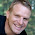Explain about the Macroscopic view and microscopic view

Macroscopic view:
• In this system the analysis is done by measuring properties like pressure, Temperature etc..
• It does not explain about the mater of structure
• By the help of gauges, the properties are measured.
• In this system behave of all the molecules are equal.
• It takes less time for measuring the properties.
• In this system, by using few co-ordinates are required to describe it.
• To approach for the analysis of macroscopic, it required only simple mathematical formulae.
• This type of process is known as Classical thermodynamics.

Microscopic View:

• This process is explained by the microscopic properties of matter correlating with molecular   configuration and
•  Intermolecular forces.
• This system deals with the thermodynamic system of time average.
• In this system, the molecules have its own properties and the velocity is independent.
• To describe this system it required large number of molecules.
• It is hard to measure the variables which are used to describe the system.
• It takes more time to measure the variables.
• To describe this system it required both mathematical and statistical methods.
• This type of process is known as statistical thermodynamics.
1.1.